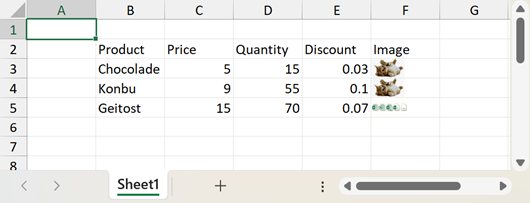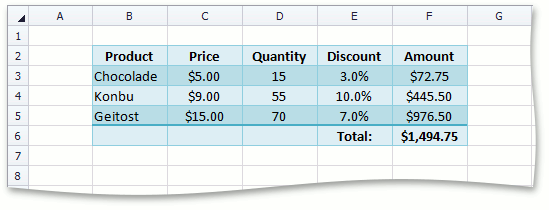.NET Framework 4.5.2+

# TableCollection Interface

A collection of tables contained in a worksheet.

## Declaration

``````public interface TableCollection :
ISimpleCollection<Table>,
IEnumerable<Table>,
IEnumerable,
ICollection``````

## Remarks

The TableCollection collection contains Table objects and is available via the Worksheet.Tables property. To add a new table to the collection, use the TableCollection.Add method. To delete existing tables, use the Table.Delete, TableCollection.Remove, TableCollection.RemoveAt or TableCollection.Clear methods.

## Example

This example demonstrates how to create a table from a range of cells. The sample range includes a list of products and invoice information on each product: price, quantity and discount.The resulting table will provide an additional column to calculate the amount per product, and an additional row to show the total amount. Follow the steps below:

1. Create a Table

Add a new Table object to the worksheet’s collection of tables (Worksheet.Tables) via the TableCollection.Add method. Pass the following parameters:

• The range of cells that you wish to format as a table. Use the “B2:F5” range reference instead of “B2:E5” to include an additional column to the right that calculates and displays product amounts.
• A Boolean value indicating that the top row of the specified range will be the table header.
2. Format the Table

Format the table by applying one of the built-in table styles. To do this, set the Table.Style property to the table style object from the IWorkbook.TableStyles collection. Access the desired style by its BuiltInTableStyleId identifier.

3. Table Columns

Access table columns by their indexes from the column collection that the Table.Columns property returns.

To specify the last column header, set its TableColumn.Name property to “Amount”. Headers of other table columns are automatically set to the values of the corresponding cells:

• table.Columns.Name = “Product”
• table.Columns.Name = “Price”
• table.Columns.Name = “Quantity”
• table.Columns.Name = “Discount”
5. Calculated Column

Specify the formula to calculate the product amount, and assign it to the Amount column using the TableColumn.Formula property. In the formula, refer to table columns by their headers.

6. Table Total Row

Set the Table.ShowTotals property to true, to display the total row at the bottom of the table.

7. Total Row Function

Specify the function to calculate the total amount. To do this, set the TableColumn.TotalRowFunction property of the Amount column to TotalRowFunction.Sum.

Note

In the total row, you can use any formulas you wish, not only functions listed by the TotalRowFunction enumerator. To use a custom formula in the total row, assign it to the TableColumn.TotalRowFormula property of the required table column.

8. Table Ranges and Data Ranges

Specify number formats to display numbers as currency values in the Price and Amount columns, and as percentage values in the Discount column. To access the data range of a table column, use the TableColumn.DataRange property.

Use the Table.HeaderRowRange and Table.TotalRowRange properties to access table header and total row ranges, and set the alignment.

Change the width of table columns. To do this, access the table range via the Table.Range property, and use its CellRange.ColumnWidthInCharacters property.

9. The image below shows the results.``````' Insert a table in the worksheet.
Dim table As Table = worksheet.Tables.Add(worksheet("B2:F5"), True)

' Format the table by applying a built-in table style.
table.Style = workbook.TableStyles(BuiltInTableStyleId.TableStyleMedium27)

' Access table columns and name them.
Dim productColumn As TableColumn = table.Columns(0)
productColumn.Name = "Product"
Dim priceColumn As TableColumn = table.Columns(1)
priceColumn.Name = "Price"
Dim quantityColumn As TableColumn = table.Columns(2)
quantityColumn.Name = "Quantity"
Dim discountColumn As TableColumn = table.Columns(3)
discountColumn.Name = "Discount"
Dim amountColumn As TableColumn = table.Columns(4)
amountColumn.Name = "Amount"

' Set the formula to calculate the amount per product
' and display results in the "Amount" column.
amountColumn.Formula = "=[Price]*[Quantity]*(1-[Discount])"

' Display the total row in the table.
table.ShowTotals = True

' Set the label and function to display the sum of the "Amount" column.
discountColumn.TotalRowLabel = "Total:"
amountColumn.TotalRowFunction = TotalRowFunction.Sum

' Specify the number format for each column.
priceColumn.DataRange.NumberFormat = "\$#,##0.00"
discountColumn.DataRange.NumberFormat = "0.0%"
amountColumn.Range.NumberFormat = "\$#,##0.00;\$#,##0.00;"""";@"

' Specify horizontal alignment for header and total rows of the table.